Search

The Remainder TheoremThe Remainder Theorem is useful for evaluating polynomials at a given value of x, though it might not seem so, at least at first blush. This is because the tool is presented as a theorem with a proof, and you probably don't feel ready for proofs at this stage in your studies. Fortunately, you don't "have" to understand the proof of the Theorem; you just need to understand how to use the Theorem.

The Remainder Theorem starts with an unnamed polynomial p(x), where "p(x)" just means "some polynomial p whose variable is x". Then the Theorem talks about dividing that polynomial by some linear factor x  a, where a is just some number. Then, as a result of the long polynomial division, you end up with some polynomial answer q(x) (the "q" standing for "the quotient polynomial") and some polynomial remainder r(x).

As a concrete example of p, aq, and r, let's look at the polynomial p(x) = x3  7x  6, and let's divide by the linear factor x  4 (so a = 4):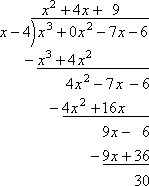So we get a quotient of q(x) = x2 + 4x + 9 on top, with a remainder of r(x) = 30.

You know, from long division of regular numbers, that your remainder (if there is one) has to be smaller than whatever you divided by. In polynomial terms, since we're dividing by a linear factor (that is, a factor in which the degree on x is just an understood "1"), then the remainder must be a constant value. That is, when you divide by "x  a", your remainder will just be some number.

The Remainder Theorem then points out the connection between division and multiplication. For instance, since 12 ÷ 3 = 4, then 4 × 3 = 12. If you get a remainder, you do the multiplication and then add the remainder back in. For instance, since 13 ÷ 5 = 2 R 3, then 13 = 5 × 2 + 3. This process works the same way with polynomials. That is:

If   p(x) / (x  a)  =  q(x)  with remainder  r(x),

then  p(x)  =  (x  a) q(x)  +  r(x).

(Technically, this "if - then" statement is the "Division Algorithm for Polynomials". But the Algorithm is the basis for the Remainder Theorem.)

In terms of our concrete example: Copyright © Elizabeth Stapel 2002-2011 All Rights Reserved

Since  (x^3  7x  6) / (x  4)   =   x2 + 4x + 9   with remainder 30,

then   x3  7x  6  =   (x  4) (x2 + 4x + 9)  +  30.

The Remainder Theorem says that we can restate the polynomial in terms of the divisor, and then evaluate the polynomial at x = a. But when x = a, the factor "x  a" is just zero! Then evaluating the polynomial at x = a gives us:

Advertisement

p(a) = (a  a)q(a) + r(a)
= (0)q(a) + r(a)
= 0 + r(a)
= r(a)

But remember that the remainder term r(a) is just a number! So the value of the polynomial p(x) at
x = a is the same as the remainder you get when you divide that polynomial p(x) by x  a. In terms of our concrete example:

p(4) = (4  4)((4)2 + 4(4) + 9) + 30
= (0)(16 + 16 + 9) + 30
= 0 + 30
= 30

But you gotta think: Okay, fine; the value of the polynomial p(x) at x = a is the remainder r(a) when you divide by x  a, but who wants to do the long division each time you have to evaluate a polynomial at a given value of x?!? You're right; this would be overkill. Fortunately, that's not what they really want you to do.

When you are dividing by a linear factor, you don't "have" to use long polynomial division; instead, you can use synthetic division, which is much quicker. In our example, we would get: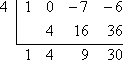Note that the last entry in the bottom row is 30, the remainder from the long division (as expected) and also the value of  p(x) = x3  7x  6 at x = 4. And that is the point of the Remainder Theorem: There is a simpler, quicker way to evaluate a polynomial p(x) at a given value of x, and this simpler way is not to evaluate p(x) at all, but to instead do the synthetic division at that same value of x. Here are some examples:

• Use the Remainder Theorem to evaluate  f (x) = 6x3  5x2 + 4x  17 at x = 3.

First off, even though the Remainder Theorem refers to the polynomial and to long division and to restating the polynomial in terms of a quotient, a divisor, and a remainder, that's not actually what I'm meant to be doing. Instead, I'm supposed to be doing synthetic division, using "3" as the divisor: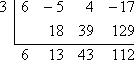Since the remainder (the last entry in the bottom row) is 112, then the Remainder Theorem says that:

f (3) = 112.

• Using the Remainder Theorem, find the value of f (5), for   f (x) = 3x4 + 2x3 + 4x.

I need to do the synthetic division, remembering to put zeroes in for the powers of x that are not included in the polynomial: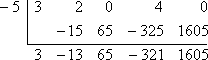Since the remainder is 1605, then, thanks to the Remainder Theorem, I know that:

f (5) = 1605.

• Use the Remainder Theorem to determine whether x = 2 is a zero of

f (x) = 3x7  x4 + 2x3  5x2  4

For x = 2 to be a zero of  f (x), then  f (2) must evaluate to zero. In the context of the Remainder Theorem, this means that my remainder, when dividing by x = 2, must be zero: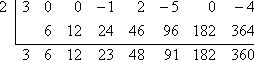The remainder is not zero. Then  x = 2 is not a zero of f (x).

• Use the Remainder Theorem to determine whether x = 4 is a solution of

x6 + 5x5 + 5x4 + 5x3 + 2x2  10x  8 = 0

For x = 4 to be a solution of f (x) = x6 + 5x5 + 5x4 + 5x3 + 2x2  10x  8 = 0, it must be that  f (4) = 0. In the context of the Remainder Theorem, this means that the remainder, when dividing by x = 4, must be zero: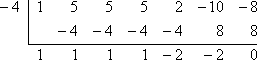The remainder is zero. Then x = 4 is a solution of the given equation.

Top  |  Return to Index

 Cite this article as: Stapel, Elizabeth. "The Remainder Theorem." Purplemath. Available from     https://www.purplemath.com/modules/remaindr.htm. Accessed [Date] [Month] 2016

Study Skills Survey

Tutoring from Purplemath
Find a local math tutor

 Copyright © 2021  Elizabeth Stapel   |   About   |   Terms of Use   |   Linking   |   Site Licensing Contact Us